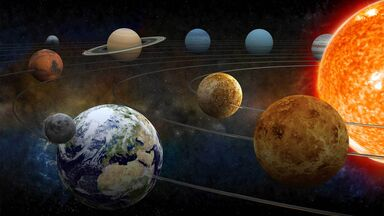# Examples of Mass

The term "mass" is used to refer to the amount of matter in any given object. Mass is often confused with weight; however, weight is a reference that relies on gravity in its determination. For instance, a person or object may be weightless on the moon because of the lack of gravity, but that same person or object maintains the same mass regardless of location. Keep reading for more examples of mass both on Earth and in our solar system.planets orbiting the solar system

## Mass of Everyday Objects

Mass is measured in kilograms (kg) to determine how much matter something contains. Scientists use the same measurement for very small items and enormous items. Take a look around your house to see if you can guess — or measure — their mass.

Take a look at these examples of mass in everyday objects.

 Everyday Object Mass sheet of paper 0.0045 kg pencil 0.0085 kg cell phone 0.141 kg book 0.34 kg bicycle 11.3 kg dog 18 kg average child 22 kg average adult 77 kg horse 771 kg car 2,000 kg house 45,359 kg

The mass of these objects might match their weight — on Earth. But if you took them to a planet where the gravitational pull was different, their mass and weight wouldn't be the same anymore. The mass values would stay the same while their weight would go down, because weight depends on gravitational pull.

## Mass of Very Large Objects

When you think of the word "massive," you certainly think of an item that is very large. But once you start thinking about measuring mass, you'll find that "massive" items have quite a lot of mass. Here are the mass measurements of very large, massive objects on Earth.

 Large Object Mass big rig 36,287 kg airplane 40,823 kg military tank 54,531 kg space shuttle (empty) 74,842 kg space shuttle (loaded) 2,000,000 kg Titanic ship (1912) 47,454,833 kg cruise ship (modern) 231,679,560 kg skyscraper 317,514,659 kg

## Mass of Solar Objects

If you're still confused about measuring weight vs. mass, take it to the sky. Planets, stars, moons, and other celestial objects may defy the laws of Earth gravity, but that doesn't mean they're without mass. Here are some examples of the mass of solar objects and their type.

 Solar Object Mass Sol (sun) 1.9891 x 1030 kg Jupiter (planet) 1.899 x 1027 kg Saturn (planet) 5.6846 x 1026 kg Neptune (planet) 1.0243 x 1026 kg Uranus (planet) 8.6832 x 1025 kg Earth (planet) 5.9736 x 1024 kg Venus (planet) 4.8685 x 1024 kg Mars (planet) 6.4185 x 1023 kg Mercury (planet) 3.302 x 1023 kg Luna (moon of Earth) 7.349 x 1022 kg Ganymede (moon of Jupiter) 1.482 x 1023 kg Callisto (moon of Jupiter) 1.076 x 1023 kg Io (moon of Jupiter) 8.94 x 1022 kg Europa (moon of Jupiter) 4.80 x 1022 kg Titan (moon of Saturn) 1.345 x 1023 kg Rhea (moon of Saturn) 2.3166 x 1021 kg Iapetus (moon of Saturn) 1.9739 x 1021 kg Hyperion (moon of Saturn) 1.08 x 1019 kg Ariel (moon of Uranus) 1.35 x 1021 kg Triton (moon of Neptune) 2.147 x 1022 kg Pluto (dwarf planet) 1.305 x 1022 kg 65 Cybele (asteroid) ~1.15 x 1019 kg 7 Iris (asteroid) 1.0 x 1019 kg 324 Bamberga (asteroid) 1.0 x 1019 kg

These are all examples of different solar objects along with the mass of each object. Every object has its own unique amount of matter and thus has its own mass.

## How to Find an Object's Mass

Finding an object's mass isn't as easy as placing it on a scale. How would we ever be able to measure planets or asteroids if that was all it took?

You find an object's with the following equations:

• mass = volume x density (m = v x d)
• mass = weight / gravity (m = w/g)

Once you find an object's mass, you can find its weight (measured in newtons) by multiplying the mass by the value of the gravitational field (g = 9.8 m/s2 on Earth). When you measure something on a scale, this conversion is done for you. Even this equation shows that mass does not require gravity as part of its calculation, while weight does.

### Mass and Energy

Mass is considered to be the equivalent of the energy content of the object. There is a famous equation created by Albert Einstein that allows you to convert mass to energy content.

The equation is: E=mc2

In this equation, E= energy, m = mass and C= the speed of light. If you know the energy of an object, or its mass and the speed of light, you can then use this equation to determine the missing element in the equation.

## Physical Properties of Science

Knowledge of mass is crucial in science. It tells you how much space an item takes up in the universe. Learn more about the physical properties of matter, including volume, length and area. Or, if you're ready for some change, take a look at 50 everyday examples of physical changes in the world.